# ₦airaland Forum

Welcome, Guest: Join Nairaland / LOGIN! / Trending / Recent / New
Stats: 2,209,523 members, 4,821,911 topics. Date: Tuesday, 19 March 2019 at 12:50 AM

## What Is The Total Weights Of The Animals In This Picture? - Education - Nairaland

(1) (2) (3) (4) (Reply) (Go Down)

 What Is The Total Weights Of The Animals In This Picture? by Folksyharry(m): 7:00am On Feb 13, 2015 what will the three animals weigh? 5 Likes 4 Shares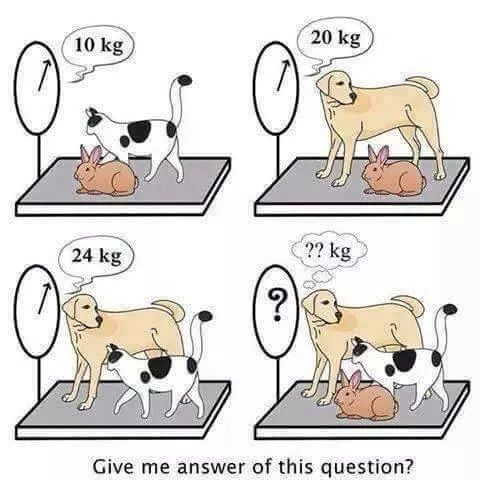Re: What Is The Total Weights Of The Animals In This Picture? by a4jasper(m): 7:13am On Feb 13, 2015 Combined weight is 27kg......i.e. The cat is 7kg, Rabbit 3kg & the Dog is 17kg 71 Likes 5 Shares Re: What Is The Total Weights Of The Animals In This Picture? by Beamborla(f): 7:23am On Feb 13, 2015 C-7D-17R-3=27 34 Likes 1 Share Re: What Is The Total Weights Of The Animals In This Picture? by EmmySparky(m): 10:26am On Feb 13, 2015 D rabbit is 3 the cat is 7 while the dog is 17..combined they are 27kg 10 Likes Re: What Is The Total Weights Of The Animals In This Picture? by laivwire(m): 12:24pm On Feb 13, 2015 It is 31 ohok, it is 27 Re: What Is The Total Weights Of The Animals In This Picture? by Nobody: 12:24pm On Feb 13, 2015 SIMPLE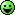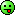1 Like 1 Share Re: What Is The Total Weights Of The Animals In This Picture? by Nobody: 12:25pm On Feb 13, 2015 laivwire:It is 31 ohYOU DULL SMALL SHA7 Likes Re: What Is The Total Weights Of The Animals In This Picture? by husseinjnr: 12:31pm On Feb 13, 2015 Let A= Rabbit B= Cat C = dogFrom Figure above, in simple analysis A + B = 10kg -----------equation 1A + C = 20kg -----------equation 2B + C = 24 kg ---------- equation 3From equation 2, A= 20- CFrom equation 3B= 24- CSubstitute A and B into equation 120-C + 24-C =1044-2C = 10-2C= 10-44-2C = -34C= 34/2C= 17Substitute C into equation 2A+ 17 = 20A= 3Substitute C into equation 3B+17 = 24B= 7ThereforeA= 3, B= 7, C= 17Total weight is 3+7+17 = 27Rabbit= 3kgCat = 7kgDog = 17kg 357 Likes 27 Shares Re: What Is The Total Weights Of The Animals In This Picture? by Nobody: 12:32pm On Feb 13, 2015 How are mine supposed to know when it's just a drawing?bring the animals in real life and I'll weigh them simple!! 20 Likes 1 Share Re: What Is The Total Weights Of The Animals In This Picture? by laivwire(m): 12:39pm On Feb 13, 2015 jacksparrow1207:YOU DULL SMALL SHAlol, Didnt calculate the Rabbit. Assumed the Rabbit and Cat were both 7kg. Re: What Is The Total Weights Of The Animals In This Picture? by Nobody: 12:56pm On Feb 13, 2015 laivwire:lol, Didnt calculate the Rabbit. Assumed the Rabbit and Cat were both 7kg. LOL.Re: What Is The Total Weights Of The Animals In This Picture? by lifestyle1(m): 1:25pm On Feb 13, 2015 Lets assume rabbit, cat and dog’s weight are R,C and D correspondingly.then we can sayEquation 1: R + C = 10Equation 2: D + R = 20Equation 3: C + D= 24Then we need to find value of C + R + DOn adding Equation 1,2 and 32R + 2 C + 2D = 54 => R+C+D = 27Na expo i use o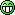25 Likes Re: What Is The Total Weights Of The Animals In This Picture? by 5p1naz(m): 1:25pm On Feb 13, 2015 put a \$ sign before those figures and watch me give you an answer in miliseconds.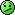7 Likes Re: What Is The Total Weights Of The Animals In This Picture? by kessluvall(f): 1:25pm On Feb 13, 2015 To me, a Dog, a Cat and a Rabbit cannot enter the same Scale.The answer is zero-0.Lol.=27 7 Likes Re: What Is The Total Weights Of The Animals In This Picture? by apoti(m): 1:25pm On Feb 13, 2015 27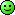Re: What Is The Total Weights Of The Animals In This Picture? by iiichidodo: 1:25pm On Feb 13, 2015 Mi tink dem ah anchor 37kg ca de dog an cat an rabbit fi equal,ya no... Re: What Is The Total Weights Of The Animals In This Picture? by Babyboy1986(m): 1:25pm On Feb 13, 2015 Make i google am now 1 Like Re: What Is The Total Weights Of The Animals In This Picture? by Sierraloaded: 1:26pm On Feb 13, 2015 HmmmDailyTimes:ALSO READ: Watch & Download: When Love Dies - Nigerian Nollywood Movie Re: What Is The Total Weights Of The Animals In This Picture? by Tenim47(m): 1:29pm On Feb 13, 2015 Am here to learn Re: What Is The Total Weights Of The Animals In This Picture? by WhiZTiM(m): 1:29pm On Feb 13, 2015 Hold on... Lemme ask Prof. Maurice Iwu Re: What Is The Total Weights Of The Animals In This Picture? by Hysmady(m): 1:29pm On Feb 13, 2015 Cat 7Rabbit 3Dog 17 Re: What Is The Total Weights Of The Animals In This Picture? by jnrbayano(m): 1:30pm On Feb 13, 2015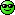Re: What Is The Total Weights Of The Animals In This Picture? by omanifrank(m): 1:30pm On Feb 13, 2015 oh mine Re: What Is The Total Weights Of The Animals In This Picture? by mcfynest(m): 1:30pm On Feb 13, 2015 husseinjnr:Let A= Rabbit B= Cat C = dogFrom Figure above, in simple analysis A + B = 10kg -----------equation 1A + C = 20kg -----------equation 2B + C = 24 kg ---------- equation 3From equation 2, A= 20- CFrom equation 3B= 24- CSubstitute A and B into equation 120-C + 24-C =1044-2C = 10-2C= 10-44-2C = -34C= 34/2C= 17Substitute C into equation 2A+ 17 = 20A= 3Substitute C into equation 3B+17 = 24B= 7ThereforeA= 3, B= 7, C= 17Total weight is 3+7+17 = 27Rabbit= 3kgCat = 7kgDog = 17kgahn ahn wat apen na 7 Likes Re: What Is The Total Weights Of The Animals In This Picture? by loomer: 1:30pm On Feb 13, 2015 husseinjnr:Let A= Rabbit B= Cat C = dogFrom Figure above, in simple analysis A + B = 10kg -----------equation 1A + C = 20kg -----------equation 2B + C = 24 kg ---------- equation 3From equation 2, A= 20- CFrom equation 3B= 24- CSubstitute A and B into equation 120-C + 24-C =1044-2C = 10-2C= 10-44-2C = -34C= 34/2C= 17Substitute C into equation 2A+ 17 = 20A= 3Substitute C into equation 3B+17 = 24B= 7ThereforeA= 3, B= 7, C= 17Total weight is 3+7+17 = 27Rabbit= 3kgCat = 7kgDog = 17kgYOU BE TEACHER? 7 Likes Re: What Is The Total Weights Of The Animals In This Picture? by Misogynist2014(m): 1:30pm On Feb 13, 2015 R+C=10D+R=20D+C=24D=17, C=7, R=3. Ans =27 Where D is dog R= rat C=cat Re: What Is The Total Weights Of The Animals In This Picture? by maestroferddi: 1:30pm On Feb 13, 2015 husseinjnr:Let A= Rabbit B= Cat C = dogFrom Figure above, in simple analysis A + B = 10kg -----------equation 1A + C = 20kg -----------equation 2B + C = 24 kg ---------- equation 3From equation 2, A= 20- CFrom equation 3B= 24- CSubstitute A and B into equation 120-C + 24-C =1044-2C = 10-2C= 10-44-2C = -34C= 34/2C= 17Substitute C into equation 2A+ 17 = 20A= 3Substitute C into equation 3B+17 = 24B= 7ThereforeA= 3, B= 7, C= 17Total weight is 3+7+17 = 27Rabbit= 3kgCat = 7kgDog = 17kgOga you go fail Jamb o...Na so dem go collect your answer sheet as you dey try form primary school teacher.This is supposed to be mental exercise and not your over-elaboration... Re: What Is The Total Weights Of The Animals In This Picture? by kilokeys(m): 1:31pm On Feb 13, 2015 lol.. i stumbled on this q last week.. in d dead of d night. went tthrough stress solving it.. while my e-bae dechandel made a good guess using a shorter method.. i almost cried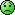3 Likes 1 Share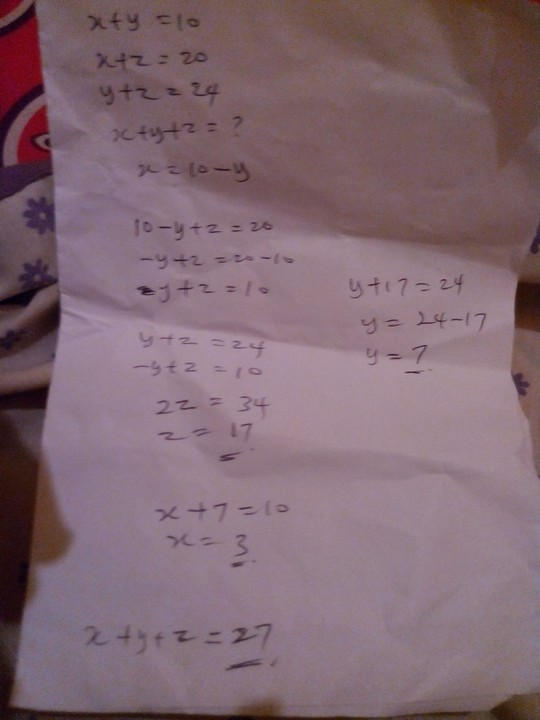Re: What Is The Total Weights Of The Animals In This Picture? by mercielizzie(f): 1:31pm On Feb 13, 2015 Lend me your calculator. 1 Like Re: What Is The Total Weights Of The Animals In This Picture? by championeh(m): 1:31pm On Feb 13, 2015 Efiko, husseinjnr:Let A= Rabbit B= Cat C = dogFrom Figure above, in simple analysis A + B = 10kg -----------equation 1A + C = 20kg -----------equation 2B + C = 24 kg ---------- equation 3From equation 2, A= 20- CFrom equation 3B= 24- CSubstitute A and B into equation 120-C + 24-C =1044-2C = 10-2C= 10-44-2C = -34C= 34/2C= 17Substitute C into equation 2A+ 17 = 20A= 3Substitute C into equation 3B+17 = 24B= 7ThereforeA= 3, B= 7, C= 17Total weight is 3+7+17 = 27Rabbit= 3kgCat = 7kgDog = 17kg Re: What Is The Total Weights Of The Animals In This Picture? by Hysmady(m): 1:31pm On Feb 13, 2015 kessluvall:To me, a Dog, a Cat and a Rabbit cannot enter the same Scale.The answer is zero-0.Lol.=27 You're funny...BtW I love ur package @ ur DP

(1) (2) (3) (4) (Reply)

(Go Up)

 Sections: politics (1) business autos (1) jobs (1) career education (1) romance computers phones travel sports fashion health religion celebs tv-movies music-radio literature webmasters programming techmarket Nairaland - Copyright © 2005 - 2019 Oluwaseun Osewa. All rights reserved. See How To Advertise. 72Disclaimer: Every Nairaland member is solely responsible for anything that he/she posts or uploads on Nairaland.